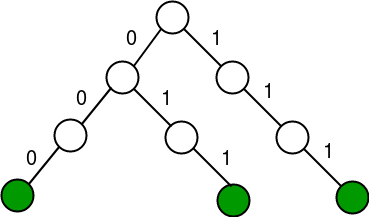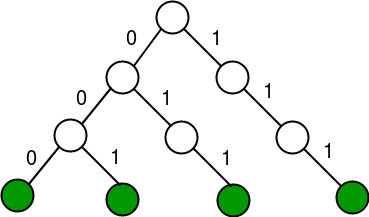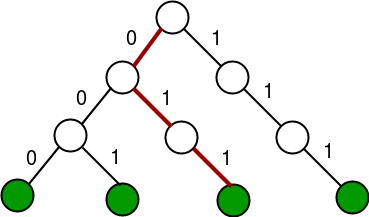# Find maximum XOR of given integer in a stream of integers

• Difficulty Level : Medium
• Last Updated : 08 Feb, 2018

You are given a number of queries Q and each query will be of the following types:

2. Query 2 : maxXOR(y) This means print the maximum possible XOR of y with all the elements already stored in the data structure.

1 <= x, y <= 10^9
1 <= 10^5 <= Q
The data structure begins with only a 0 in it.

Example:

```Input: (1 10), (1 13), (2 10), (1 9), (1 5), (2 6)
Output: 7 15

Add 10 and 13 to stream.
Find maximum XOR with 10, which is 7
Insert 9 and 5
Find maximum XOR with 6 which is 15.
```

## Recommended: Please try your approach on {IDE} first, before moving on to the solution.

A good way to solve this problem is to use a Trie. A prerequisite for this post is Trie Insert and Search.

Each Trie Node will look following:

```struct TrieNode
{
// We use binary and hence we
// need only 2 children
TrieNode* Children;
bool isLeaf;
};
```

Another thing to handle is that we have to pad the binary equivalent of each input number by a suitable number of zeros to the left before storing them. The maximum possible value of x or y is 10^9 and hence 32 bits will be sufficient.

So how does this work?
Assume we have to insert 3 and 7 into Trie. The Trie starts out with 0 and after these three insertions can be visualized like this:For simplification, the padding has been done to store each number using 3 bits. Note that in binary:
3 is 011
7 is 111
Now if we have to insert 1 into our Trie, we can note that 1 is 001 and we already have path for 00. So we make a new node for the last set bit and after connecting, we get this:Now if we have to take XOR with 5 which is 101, we note that for the leftmost bit (position 2), we can choose a 0 starting at the root and thus we go to the left. This is the position 2 and we add 2^2 to the answer.
For position 1, we have a 0 in 5 and we see that we can choose a 1 from our current node. Thus we go right and add 2^1 to the answer.
For position 0, we have a 1 in 5 and we see that we cannot choose a 0 from our current node, thus we go right.The path taken for 5 is shown above. The answer is thus 2^2 + 2^1 = 6.

 `// C++ program to find maximum XOR in``// a stream of integers``#include``using` `namespace` `std;`` ` `struct` `TrieNode``{``    ``TrieNode* children;``    ``bool` `isLeaf;``};`` ` `// This checks if the ith position in``// binary of N is a 1 or a 0``bool` `check(``int` `N, ``int` `i)``{``    ``return` `(``bool``)(N & (1<isLeaf = ``false``;``    ``temp->children = NULL;``    ``temp->children = NULL;``    ``return` `temp;``}`` ` `// Inserts x into the Trie``void` `insert(TrieNode* root, ``int` `x)``{``    ``TrieNode* Crawler = root;`` ` `    ``// padding upto 32 bits``    ``for` `(``int` `i = 31; i >= 0; i--)``    ``{``        ``int` `f = check(x, i);``        ``if` `(! Crawler->children[f])``            ``Crawler->children[f] = newNode();``        ``Crawler = Crawler->children[f];``    ``}``    ``Crawler->isLeaf = ``true``;``}`` ` `// Finds maximum XOR of x with stream of``// elements so far.``int` `query2(TrieNode *root, ``int` `x)``{``    ``TrieNode* Crawler = root;`` ` `    ``// Do XOR from root to a leaf path``    ``int` `ans = 0;``    ``for` `(``int` `i = 31; i >= 0; i--)``    ``{``        ``// Find i-th bit in x``        ``int` `f = check(x, i);`` ` `        ``// Move to the child whose XOR with f``        ``// is 1.``        ``if` `((Crawler->children[f ^ 1]))``        ``{``            ``ans = ans + (1 << i); ``// update answer``            ``Crawler = Crawler->children[f ^ 1];``        ``}`` ` `        ``// If child with XOR 1 doesn't exist``        ``else``            ``Crawler = Crawler->children[f];``    ``}`` ` `    ``return` `ans;``}`` ` `// Process x (Add x to the stream)``void` `query1(TrieNode *root, ``int` `x)``{``    ``insert(root, x);``}`` ` `// Driver code``int` `main()``{``    ``TrieNode* root = newNode();``    ``query1(root, 10);``    ``query1(root, 13);``    ``cout << query2(root, 10) << endl;``    ``query1(root, 9);``    ``query1(root, 5);``    ``cout << query2(root, 6) << endl;``    ``return` `0;``}`

```7
15
```

The space taken by the Trie is O(n*log(n)). Each query of type 1 takes O(log(n)) time. Each query of type 2 takes O(log(n)) time too. Here n is the largest query number.

Follow up problem: What if we are given three queries instead of two?